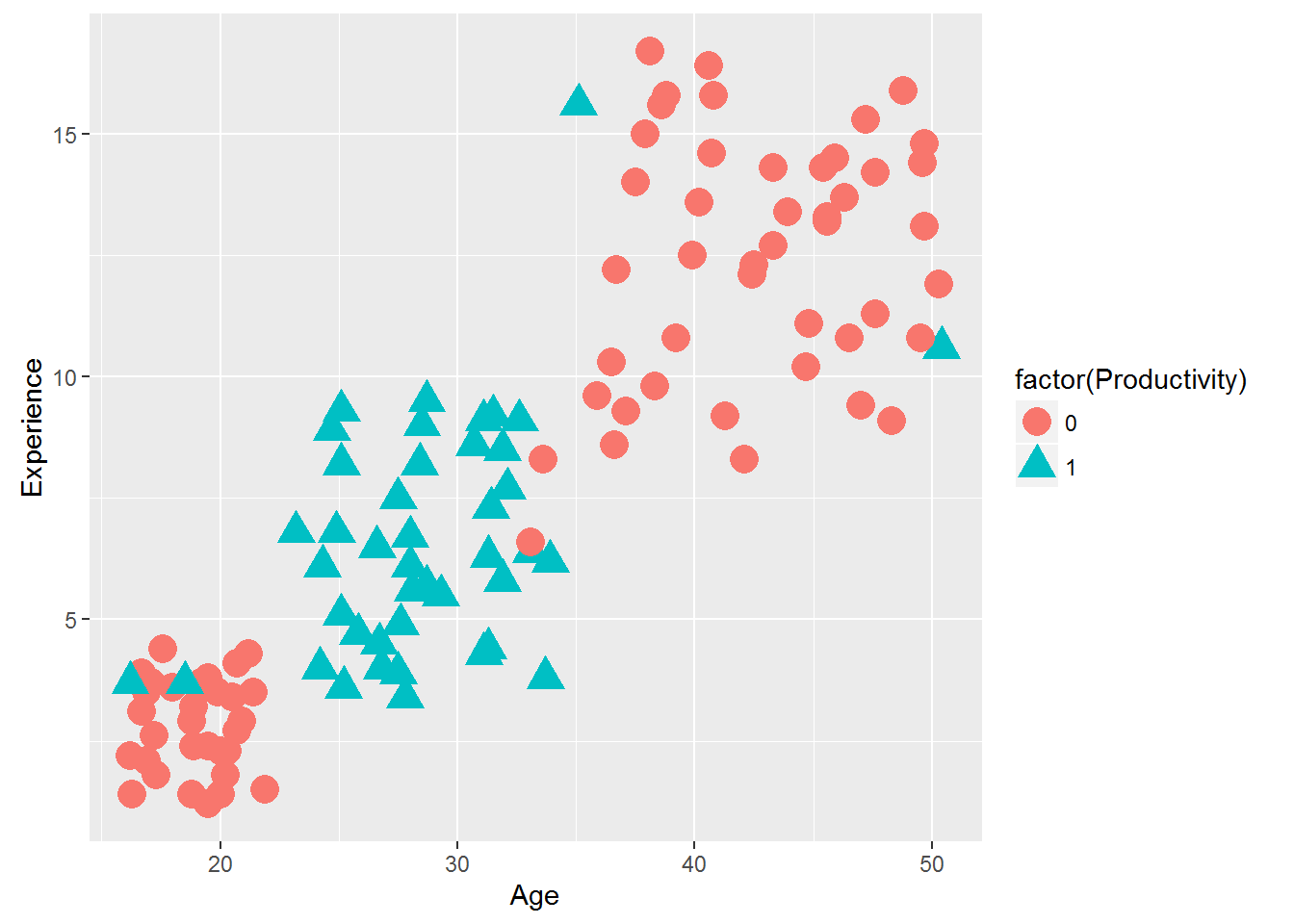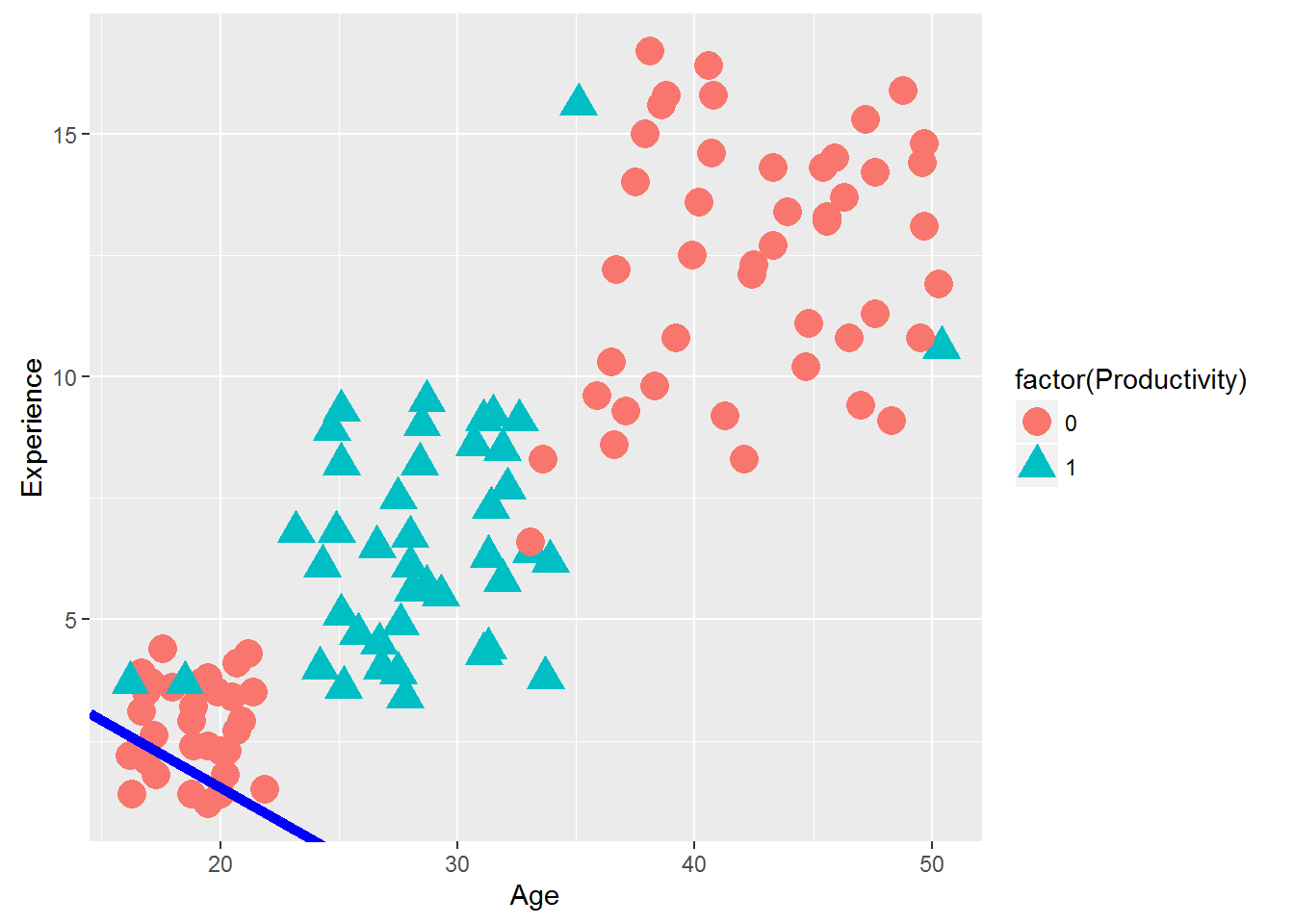• No products in the cart.

# 203.5.3 Practice : Non Linear Decision Boundary

##### Let's see what happens when we our data cannot be classified using a non linear boundary.

In previous section, we studied about Decision Boundary – Logistic Regression

Linear decision boundaries is not always way to go, as our data can have polynomial boundary too. In this post we will just see what happens if we try to use a linear function to classify a bit complex data.

`LAB: Non-Linear Decision Boundaries`
• Dataset: “Emp_Productivity/ Emp_Productivity.csv”
• Draw a scatter plot that shows Age on X axis and Experience on Y-axis. Try to distinguish the two classes with colors or shapes (visualizing the classes)
• Build a logistic regression model to predict Productivity using age and experience
• Finally draw the decision boundary for this logistic regression model
• Create the confusion matrix
• Calculate the accuracy and error rates

Here we are considering the entire data not the subset

``````####The clasification graph on overall data
library(ggplot2)
ggplot(Emp_Productivity_raw)+geom_point(aes(x=Age,y=Experience,color=factor(Productivity),shape=factor(Productivity)),size=5)````````````###Logistic Regerssion model for overall data
Emp_Productivity_logit_overall<-glm(Productivity~Age+Experience,data=Emp_Productivity_raw, family=binomial())
Emp_Productivity_logit_overall``````
``````##
## Call:  glm(formula = Productivity ~ Age + Experience, family = binomial(),
##     data = Emp_Productivity_raw)
##
## Coefficients:
## (Intercept)          Age   Experience
##     0.44784     -0.01755     -0.06324
##
## Degrees of Freedom: 118 Total (i.e. Null);  116 Residual
## Null Deviance:       155.7
## Residual Deviance: 150.5     AIC: 156.5``````
``````slope2 <- coef(Emp_Productivity_logit_overall)/(-coef(Emp_Productivity_logit_overall))
intercept2 <- coef(Emp_Productivity_logit_overall)/(-coef(Emp_Productivity_logit_overall))

####Drawing the Decision boundary

library(ggplot2)
base<-ggplot(Emp_Productivity_raw)+geom_point(aes(x=Age,y=Experience,color=factor(Productivity),shape=factor(Productivity)),size=5)
base+geom_abline(intercept = intercept2 , slope = slope2, colour = "blue", size = 2) ````````````####Accuracy of the overall model
predicted_values<-round(predict(Emp_Productivity_logit_overall,type="response"),0)
conf_matrix<-table(predicted_values,Emp_Productivity_logit_overall\$y)
conf_matrix``````
``````##
## predicted_values  0  1
##                0 69 43
##                1  7  0``````
``````accuracy<-(conf_matrix[1,1]+conf_matrix[2,2])/(sum(conf_matrix))
accuracy``````
``##  0.5798319``
21st June 2017
0 responses on "203.5.3 Practice : Non Linear Decision Boundary"